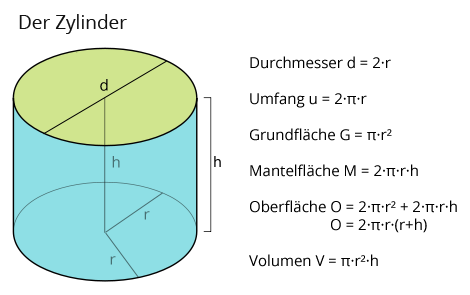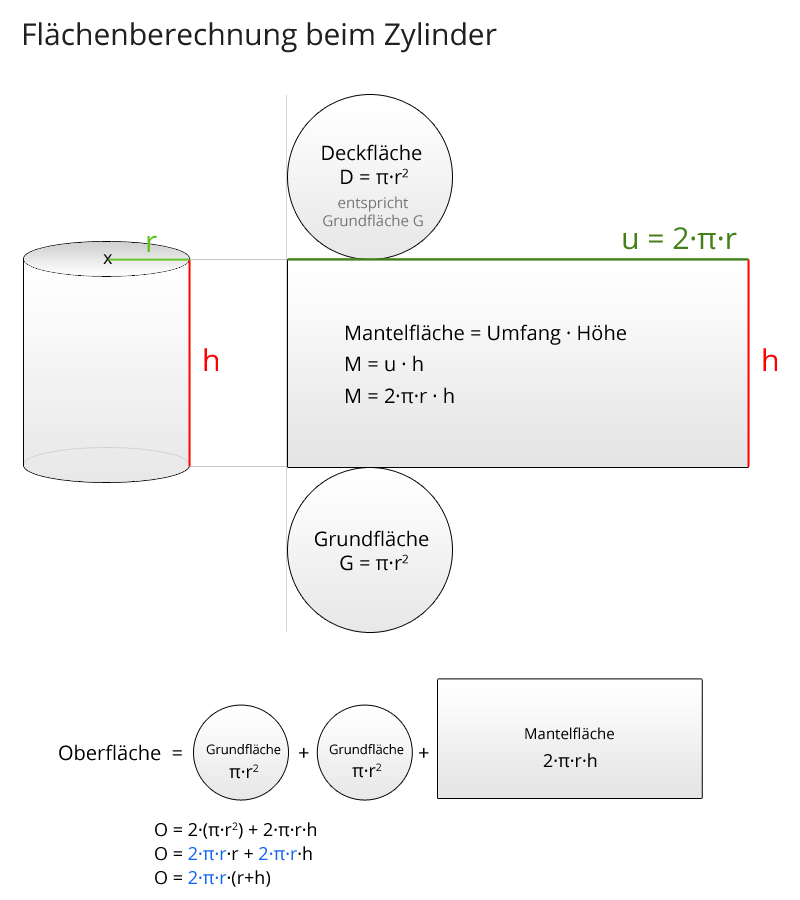# What is the circumference of a cylinder

## Calculator: cylinder

### All cylinder formulas at a glance

Here you can see the necessary formulas to calculate a cylinder:Explanations:

Diameter = 2 · radius → d = 2 · r

Circumference = 2 Pi radius → u = 2 π r

Base area = Pi · Radius² → G = π · r²

Surface area = circumference height → M = 2 π r h

Surface = 2 base area + outer surface → O = 2 π r² + 2 π r h = 2 π r (r + h)

Volume = base · height → V = π · r² · h

### What is a cylinder

Definition: A cylinder (circular cylinder) consists of two parallel opposing circular surfaces and a rectangular lateral surface that is perpendicular to the circular surfaces (base and top surface). A cylinder is a geometric body. Circular areas are of essential importance for this body, so the formulas for the circle (circular area and circumference) are required.
The following formulas apply to the circular cylinder: The circumference u is 2 · Pi · r (this is the formula for the circumference), the base area G is Pi i.e. M = u · h and thus M = 2 · Pi · r · h, the surface results from the circular area above and below (2 times the base area) and the outer surface, i.e. surface O = 2 · G + M and thus O = 2 · (π · r²) + (2 · π · r · h), whereby one often excludes the 2 · π · r and thus obtains: O = 2 · π · r · (r + h). The cylinder volume is the base times the height, i.e. V = G · h = π · r² · h. Cylinder with radius base and diameter base. Features of a cylinder

Examples from everyday life (cylinder shape):
Glass, tube, building block, motor cylinder, chair leg, pen, tablet form, etc.

#### Area calculation for the cylinder (graphic):